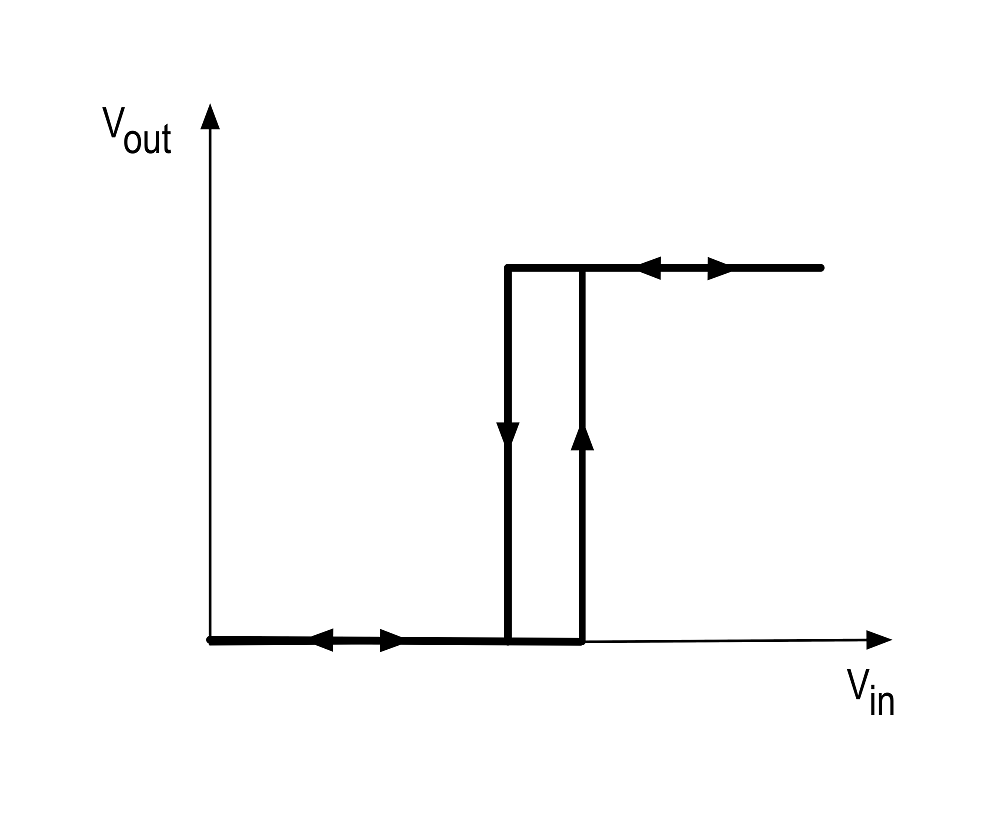# Comparator HysteresisWritten by

We are probably all familiar with comparators. A typical “inverting” comparator circuit is shown in Figure 1. Resistors R1 and R2 provide a reference voltage at the non-inverting input and the input voltage Vin is applied at the inverting input. Most comparators have open drain outputs so a pull-up resistor Rp is required at the output. Resistor Rh provides hysteresis. The output of the comparator will be high when the input voltage is lower than the voltage at the node where R1, R2 and Rh come together.FIGURE 1. The classic “inverting” comparator with hysteresis. The input impedance is high since the input is connected directly to the comparator’s inverting input.

In the absence of Rh, this voltage would be fixed by the divider formed by R1 and R2. When the input voltage is really close to this voltage the output of the comparator can oscillate between states, especially is there is any noise on the input or the supply voltage. Not very desirable. The solution is to introduce hysteresis via Rh. When Vin is low, the comparator open drain will be high impedance and the output will be pulled high by Rp. Now Rp and Rh are effectively in parallel with R1, and the voltage at the non-inverting input will be slightly higher that it was without hysteresis. Now when Vin is high the comparator output is pulled low. Now Rh is effectively in parallel with R2, slightly reducing the voltage at the non-inverting input from where it would have been without hysteresis.

The graph on the right of Figure 1 shows this graphically—we now effectively have two thresholds—a higher one when Vin is rising through the nominal threshold and a lower one when it is falling. If we make the hysteresis wider than the noise, we can eliminate any chatter or oscillation as we pass through the threshold. The equations that describe the two thresholds are below.

But what if we want a “non-inverting” comparator? We can use the circuit shown in Figure 2. This has a fixed reference at the inverting input derived from the divider formed by R1 and R2. Rin, Rh and Rp provide the hysteresis.FIGURE 2. The non-inverting version has the disadvantage that the input impedance is relatively low, and worse, it varies depending on the state of the comparator output. Any source impedance impacts the thresholds.

The formulas for this are a little trickier to calculate.

The disadvantage with this circuit compared to the “inverting” version is that the input impedance is lower and changes with comparator state. Any source impedance will form part of Rin and needs to be taken into account in calculating the hysteresis. Messy.

A different approach is to employ a MOSFET as shown in Figure 3. Vin is applied directly to the non-inverting input of the comparator, so the impedance issues go away. When the input voltage is low, the comparator output voltage is also low and the MOSFET is off. The switching threshold is set by the divider formed by R1 and R2+R3. When the input is high, the comparator output is high-impedance and Rp switches the MOSFET on, removing R3 from the divider string and reducing the switching threshold.FIGURE 3. This version of the non-inverting comparator eliminates the problems of Figure 2. It has high fixed input impedance and thresholds are unaffected by the source impedance. It comes at the cost of an additional MOSFET.

The equations for the rising and falling thresholds are easily derived.

References:

Kay, Arthur, and Timothy Claycomb. “Comparator with Hysteresis Reference Design.” Texas Instruments, 2014. https://www.ti.com/lit/ug/tidu020a/tidu020a.pdf.

“Guide to Adding Extra Hysteresis to Comparators | Maxim Integrated.” Accessed September 18, 2020. https://www.maximintegrated.com/en/design/technical-documents/app-notes/3/3616.html.

 Keep up-to-date with our FREE Weekly Newsletter! Don't miss out on upcoming issues of Circuit Cellar. Subscribe to Circuit Cellar Magazine Note: We’ve made the May 2020 issue of Circuit Cellar available as a free sample issue. In it, you’ll find a rich variety of the kinds of articles and information that exemplify a typical issue of the current magazine. Would you like to write for Circuit Cellar? We are always accepting articles/posts from the technical community. Get in touch with us and let's discuss your ideas.